# Vertical Translation

Vertical Translation

There are four types of transformation possible for a graph of a function, which are:

• Rotations
• Translations
• Reflections, and
• Scaling

Further, translation can be divided into two different types, i.e.,

• Horizontal translation
• Vertical translation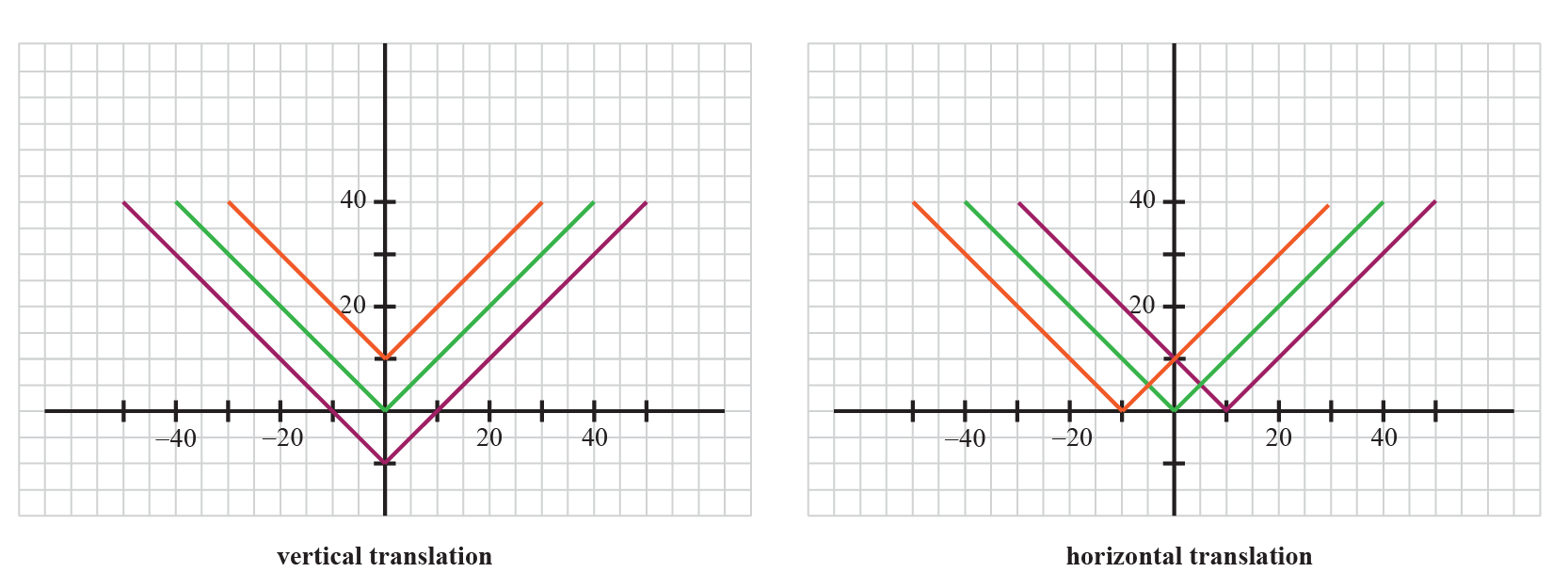In this section, we will learn about vertical translation in detail and practice solving questions around it.

You can check out the interactive simulations to know more about the lesson and try your hand at solving a few interesting interactive questions at the end of the page.

## Lesson Plan

 1 What Is a Vertical Translation? 2 Important Notes on Vertical Translation 3 Solved Examples on Vertical Translation 4 Challenging Questions on Vertical Translation 5 Interactive Questions on Vertical Translation

## What Is a Vertical Translation?

Vertical translation refers to the up or down movement of the graph of a function.

Here, the shape of the function remains the same.

It is also known as the movement/shifting of the graph along the y-axis.

In vertical translation, each point on the graph moves k units vertically and the graph is said to translated k units vertically.

The following is the graph of $$f\left( x \right) = \left| x \right|$$.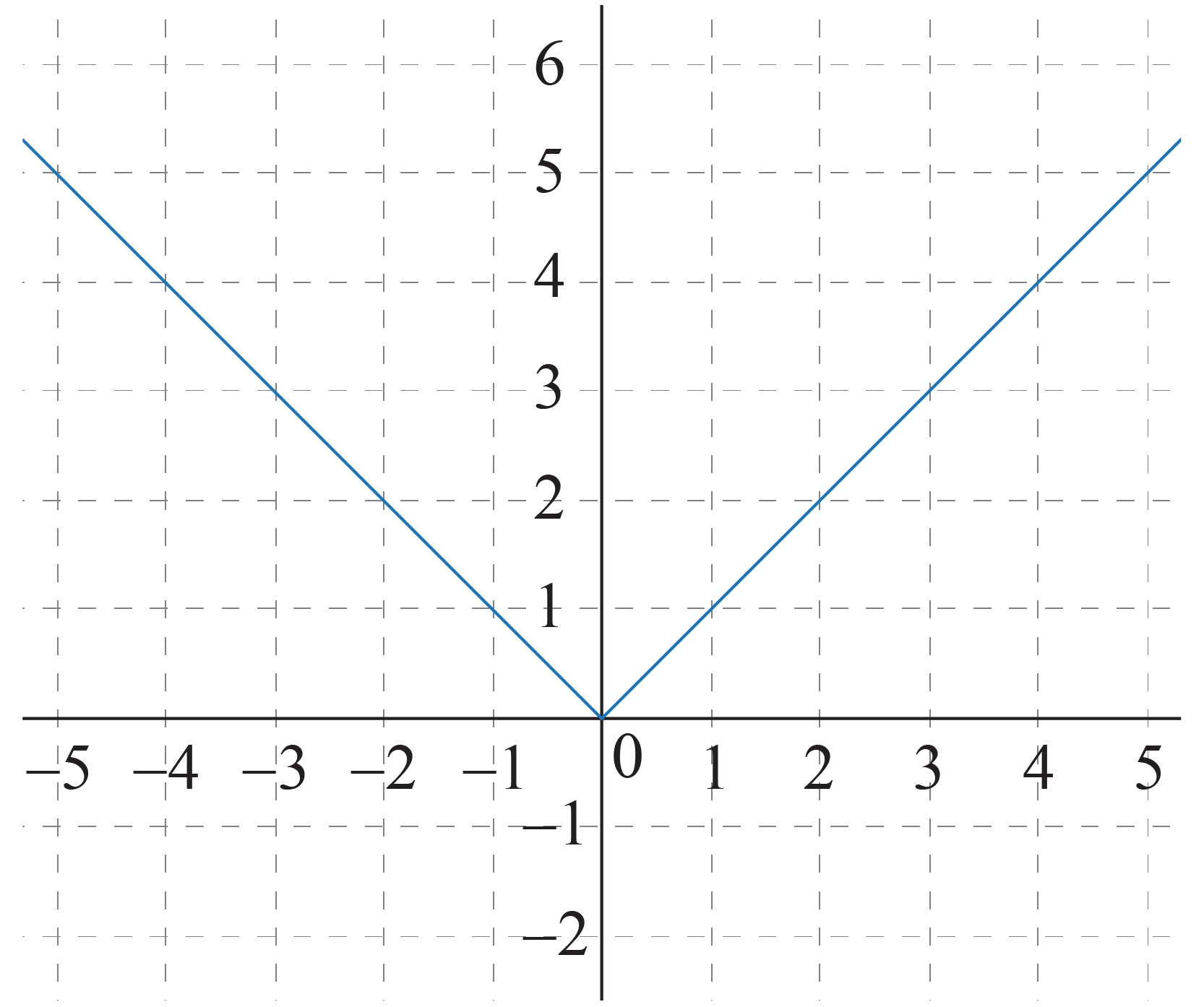Now, if we shift this exact graph 1 unit down, we will get the following graph: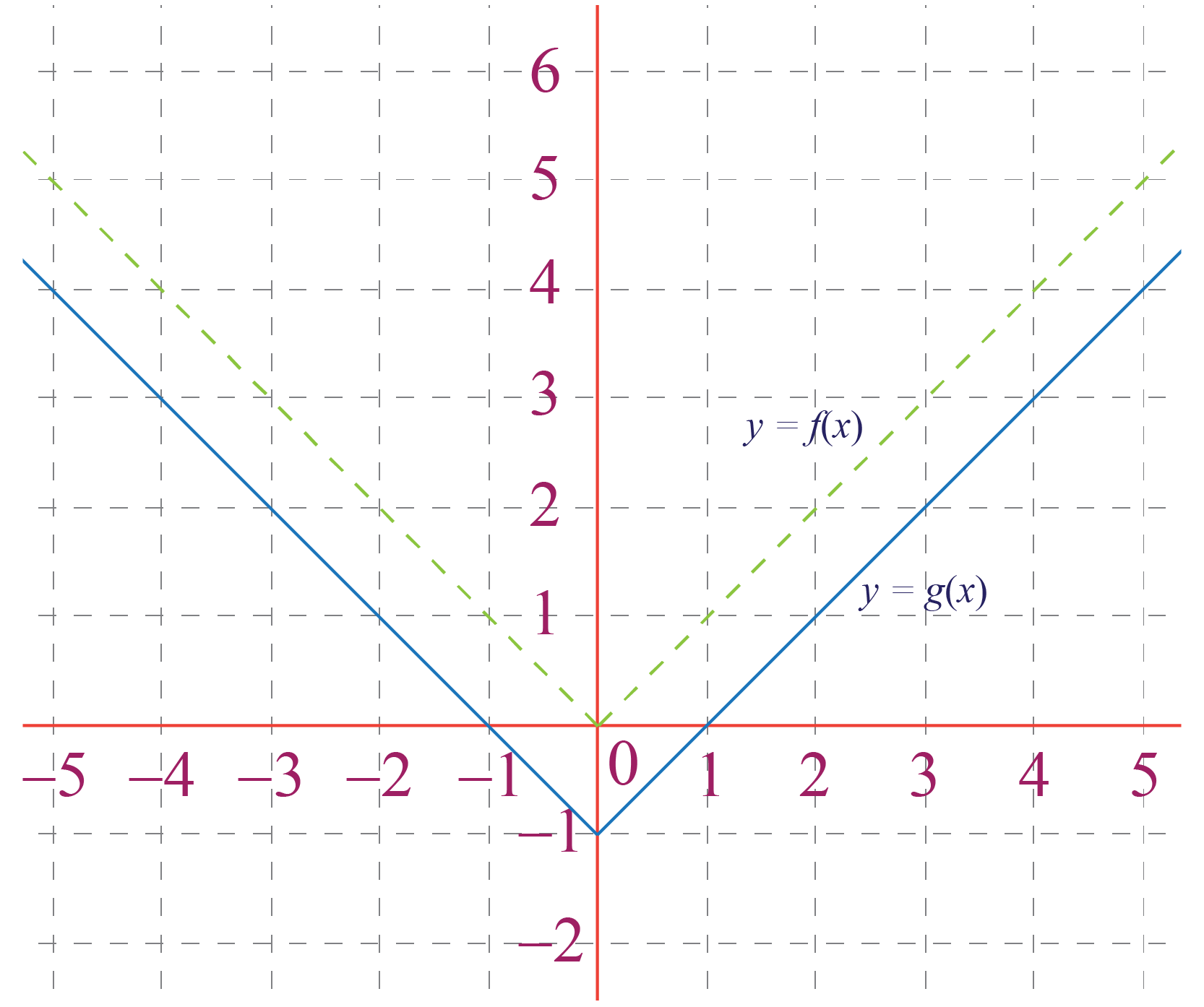This is the graph of the function $$g\left( x \right) = \left| x \right|- 1$$

This means that we can obtain the graph of g(x) by simply down-shifting the graph of f by 1 unit.

Similarly, the graph of $$h\left( x \right) = \left| x \right| + 1 = f\left( x\right) + 1$$ can be obtained by up-shifting the graph of f by 1 unit: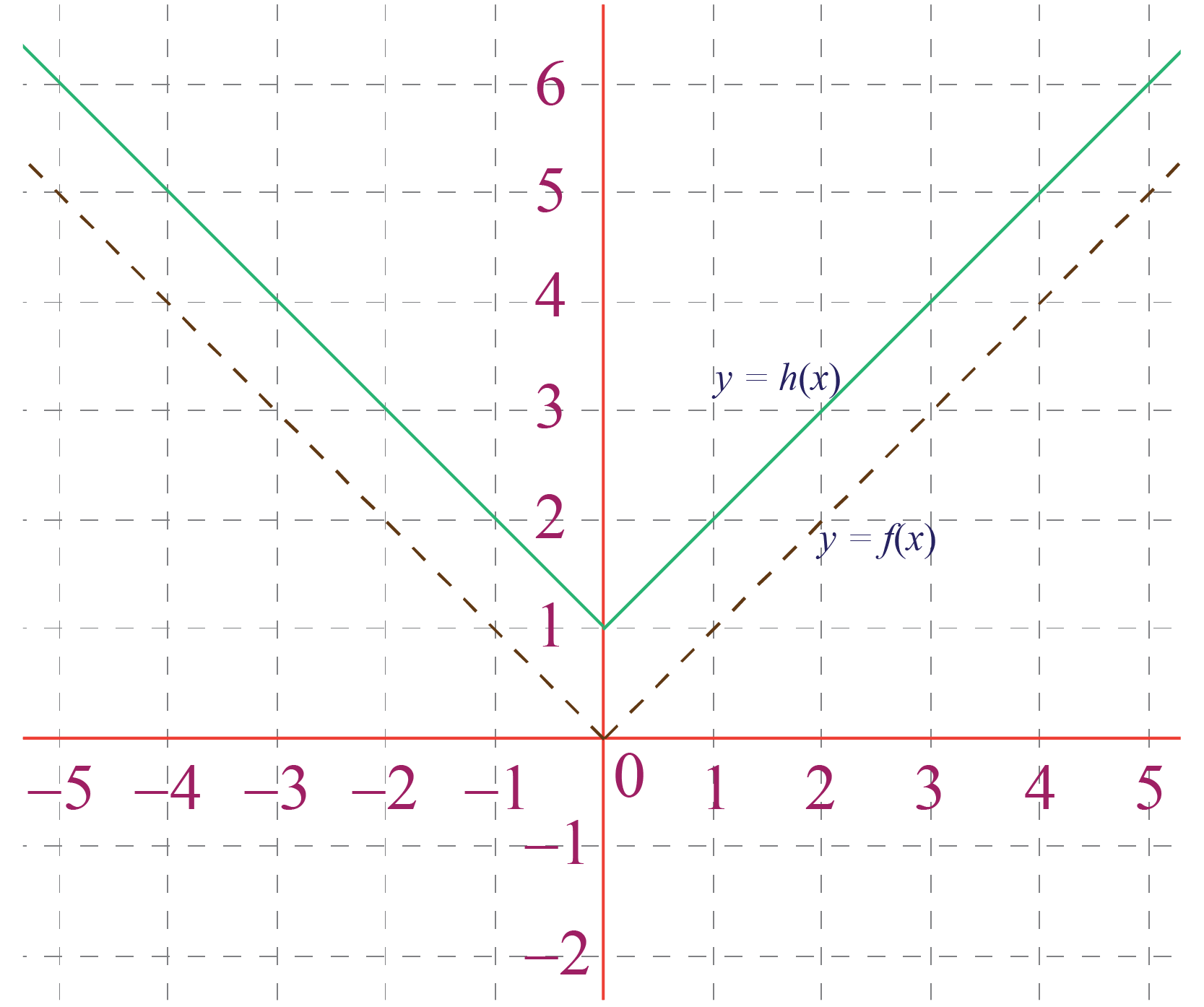Here, we have seen the vertical translation of the graph by 1 unit.

The following table shows the coordinates of the point on the different curves after translation:

The point on f(x) The point on f(x) + C The point on f(x) - C
$$(x, y)$$

$$(x, y + C)$$

$$(x, y - C)$$

Meanwhile, the shape of the function and domain of the function remains the same.

## How to Do Vertical Translation?

Let us understand this by an example:

Example

Suppose we have a basic quadratic equation $$f(x) = x^2$$ and the graphical representation of the graph is shown below.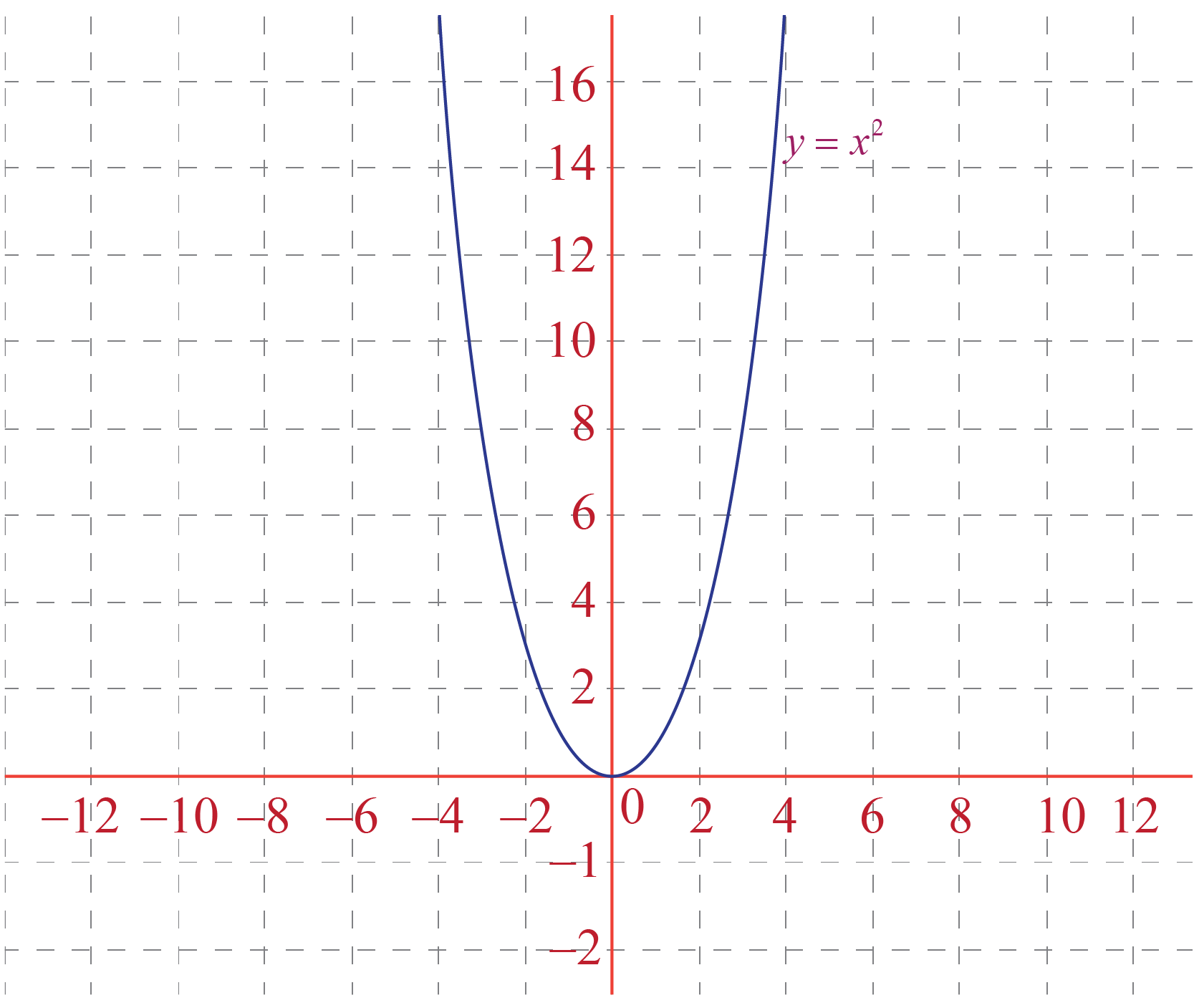Now if we want to translate this graph vertically we have to follow the given steps:

Steps

Step 1: Select the constant by which we want to translate the function.

Here we have selected $$\color{orange}{+2}$$.

Step 2: Write the new function as $$g(x) = f(x) \pm C$$, where C is the constant.

Here, our equation of the new function will be

$g(x) = f(x) \color{orange}{+2} = x^2 \color{orange}{+2}$

Step 3: Trace the basic function graph again and shift each point in the graph by $$\pm C$$, in the vertical direction( up for +C and down for -C).

Here, we have shifted the graph of $$x^2$$ by $$\color{orange}{+2}$$ units in an upward direction.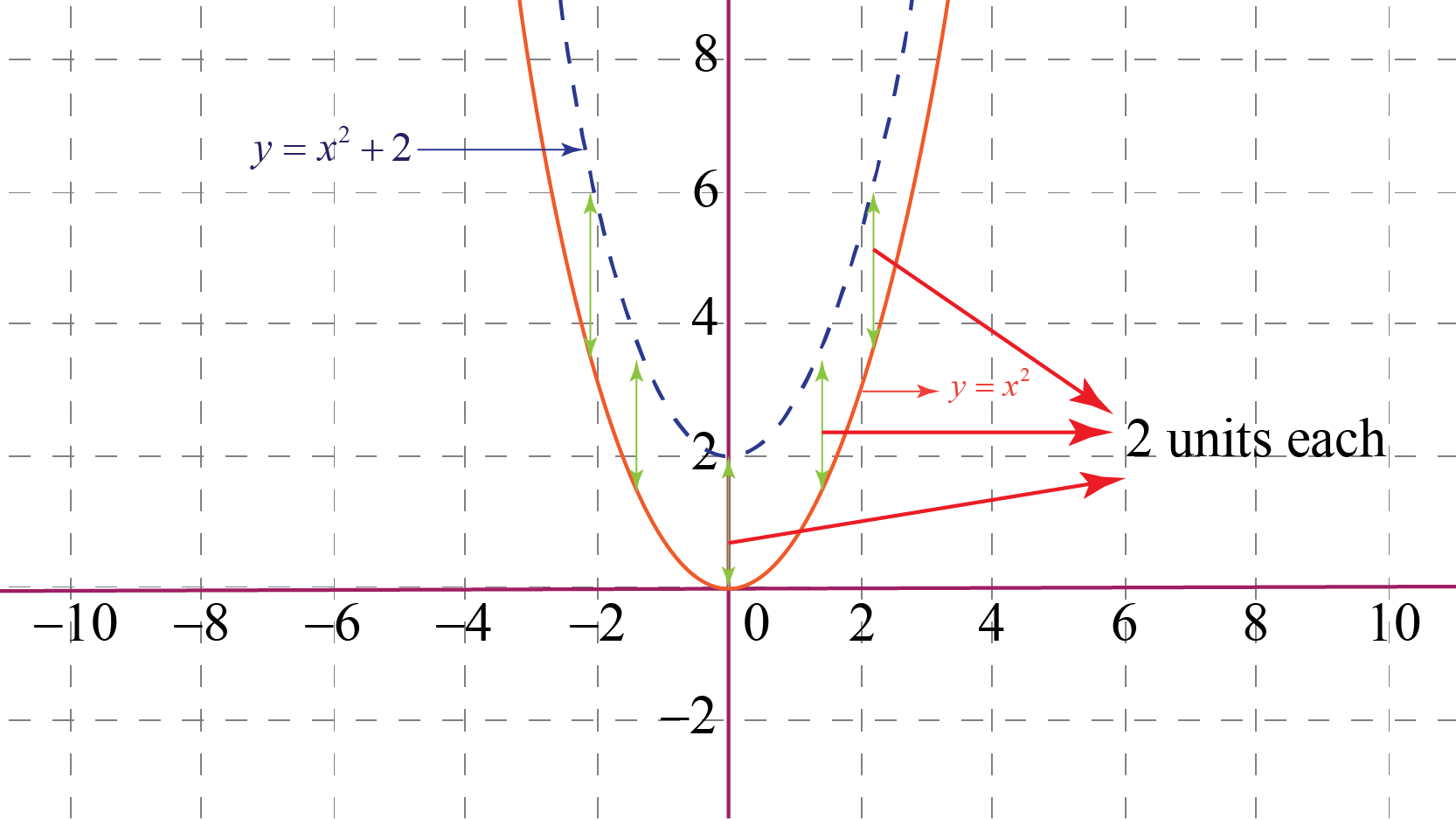Step 4: Trace the new function, this will be the vertically translated basic function by $$\pm C$$ units.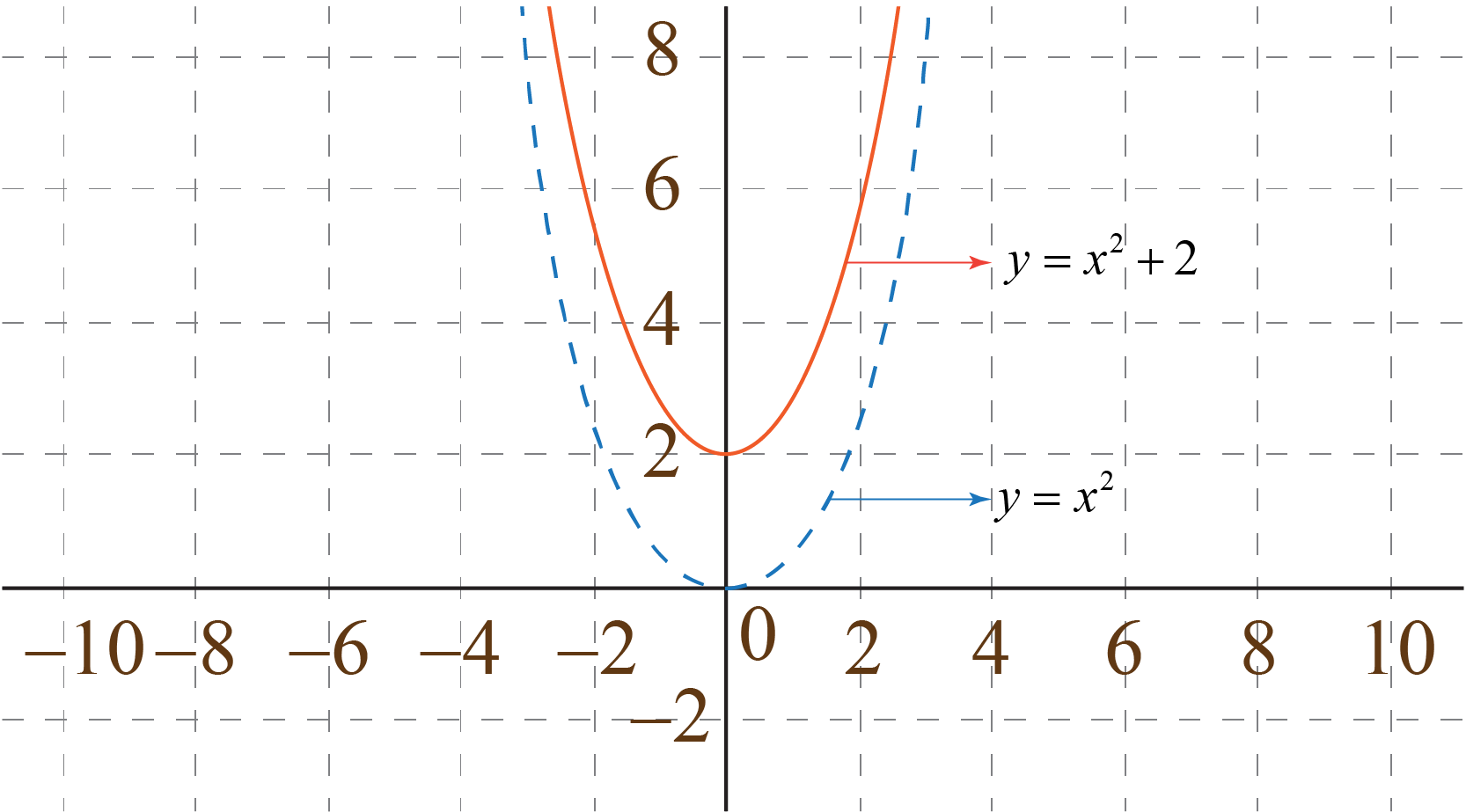Here, we have got $$\color{orange}{+2}$$ units vertically translated graph of $$x^2$$ or of the function $$g(x) = x^2 +2$$.

The domain of the function remains the same in both cases.

Try your hand at the following calculator to know more about vertical translation:

1. Vertical translation refers to the shifting of the curve along the y-axis by some specific units without changing the shape and domain of the function.
2. Vertical translation of function f(x) is given by g(x) = f(x) $$\pm$$ C.
Functions
grade 10 | Questions Set 2
Functions